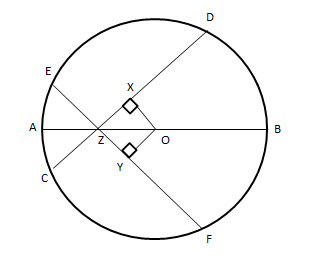If two intersecting chords of the circle make an equal angle with the diameter passing through their point of contact to the diameter, prove that the chords are equal.Verified
149.4k+ views
Hint: The chords can be proved if equal if their distance from the center is equal, which can be done by using congruency.

The figure according to the condition given in the question is shown below as,Given: Let’s suppose CD and EF are the two chords of a circle intersecting at Z. AB is the diameter which passes through their point of intersection as well. The chords are equally inclined to the diameter. It implies that$\angle XZO = \angle YZO$.
To prove: the length of the chord CD and EF are equal.

Proof:Construction: Drop two perpendiculars from center O to the chord CD and EF respectively, such that they intersect the chords at X and Y respectively.
In triangle OXZ and triangle OYZ
$\angle OXZ = \angle OYZ$ (Both are equal to ${90^o}$, as per our construction)
$\angle XZO = \angle YZO$ (Chords are equally inclined with the diameter)
$OZ = OZ$ (Both are common in the two triangles.)
Hence, $\Delta OXZ \cong \Delta OYZ$ by AAS(Angle-Angle-Side) criteria
Thus, it can be concluded that
$OX = OY$ (By CPCT- Corresponding Parts of Congruent Triangle)
Since the chords are equidistant from the center of the circle, therefore they are equal to each other.
Thus, the length of chord CD = length of chord EF.
(Proved)

Note:
The two triangles are said to be congruent if their corresponding sides and corresponding angles are equal.
There are 4 ways by which it can be proved
SSS (Side-Side-Side) Criteria
SAS (Side-Angle-Side)
AAS (Angle-Angle-Side)
ASA (Angle-Side-Angle)
AAA is not a criterion to prove the congruency of the triangle.